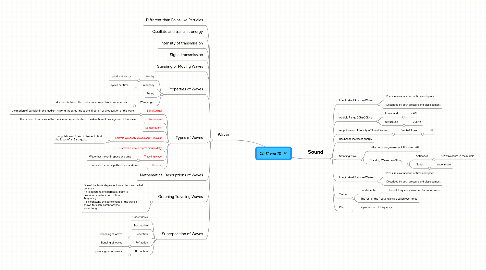# Ch 11 and 12

Just an initial demo map, so that you don't start with an empty map list ...

Jetzt loslegen. Gratis!Ch 11 and 12 von## 1. Waves

### 1.6. Properties of Waves

1.6.1. Velocity

1.6.1.1. speed and vector

1.6.2. Frequency

1.6.2.1. cycles per time

1.6.3. Period

1.6.4. Wavelength

1.6.4.1. distance between the centers of consecutive compressions

### 1.7. Types of Waves

1.7.1. Longitudinal

1.7.1.1. the motion of particles in the medium is along the same line as the direction of propagation of the wave

1.7.2. Transverse

1.7.2.1. the motion of particles in the medium is perpendicular to the direction of propagation of the wave

1.7.3. Combinations

1.7.4. Seismic Waves (S-Waves and P-Waves)

1.7.4.1. Triangulation of S- and P-Waves to find the focus of an Earthquake

1.7.5. Harmonic waves repeat sinusoidally

1.7.6. Traveling wave

1.7.6.1. Wave that moves in space and time

1.7.7. Periodic

1.7.7.1. repeats the same pattern over and over

### 1.9. Graphing Traveling Waves

1.9.1. One of the two independent variables must be held constant If x is constant, one particular point is described as a function of time. Frequency If t is constant, the basic shape of the wave is shown for each instant of time Wave Length

### 1.10. Superposition of Waves

1.10.1. Constructive

1.10.2. Destructive

1.10.3. Reflection

1.10.3.1. Bouncing of waves

1.10.4. Refraction

1.10.4.1. Bending of waves

1.10.5. Diffraction

## 2. Sound

### 2.1. Longitudinal Pressure Waves

2.1.1. Pressure as a function of time and space

2.1.2. Described by both pressure and displacement

### 2.2. Audible Range 20hz-20kHz

2.2.1. Infrasound

2.2.1.1. <20hz

2.2.2. Ultrasound

2.2.2.1. >20kHz

### 2.3. Amplitude and Intensity of Sound Waves

2.3.1. Decibel Scale

2.3.1.1. dB

### 2.5. Standing wave

2.5.1. reflecting wave, makes look like stand still

2.5.2. Standing Waves on a String

2.5.2.1. Antinodes

2.5.2.1.1. 1/2 wave lenth in the middle

2.5.2.2. Nodes

2.5.2.2.1. never move

### 2.6. Longitudinal Pressure Waves

2.6.1. Pressure as a function of time and space

2.6.2. Described by both pressure and displacement

### 2.7. Timbre

2.7.1. Fundamental

2.7.1.1. lowest frequency in a complex sound wave

2.7.2. The rest of the frequencies are called overtones

### 2.8. Pitch

2.8.1. the perception of frequency